## 30 Reputation

1 years, 137 days

## Solving Caputo fractional derivative...

Maple

Please, is there anyway I can solve below problem without replacing the Alpha with value? The error I got is "Error, (in fracdiff) Unable to determine ceiling of alpha"

>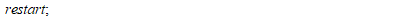>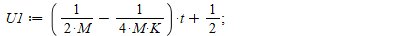>-fracdiff(U1, t, alpha)+U1/M-U1^2/(M*K)+diff(U1, t)-(diff(U1, t))/epsilon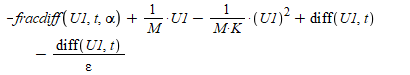>int(%, t)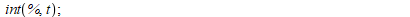## Solution of Logistic equation in Perturb...

Maple

Hi everyone,
Please I need your help, if anyone has idea of using Perturbation Theory to Solve the following Logistic Fractional Equation and ploting with iteration. Thanks

Restart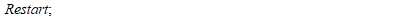u(t):=1/(m)(u(t)-(u^(2)(t))/(k));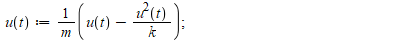NULL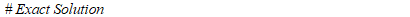uu(t):=(k*u_0)/((u_0+(u_0)*k)*(e)^(-t/(m)))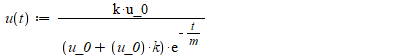NU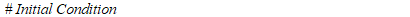LL

u(0) = u_0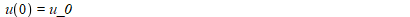NULL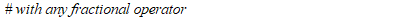## Integration problem...

Maple

Please, is there anyway I can solve this problem with a nice output? The solution I got is complicated. Please, helper is need

f := exp(-beta*x)*x^(5-alpha)

int(f, x)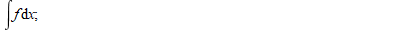beta^(alpha-6)*(-x^(-alpha)*beta^(-alpha)*(alpha^4-14*alpha^3+71*alpha^2-154*alpha+120)*(alpha-6)*(beta*x)^((1/2)*alpha)*exp(-(1/2)*beta*x)*WhittakerM(-(1/2)*alpha, -(1/2)*alpha+1/2, beta*x)/(-alpha+6)+x^(-alpha)*beta^(-alpha)*(beta^4*x^4-alpha*beta^3*x^3+alpha^2*beta^2*x^2+5*beta^3*x^3-alpha^3*beta*x-9*alpha*beta^2*x^2+alpha^4+12*alpha^2*beta*x+20*beta^2*x^2-14*alpha^3-47*alpha*beta*x+71*alpha^2+60*beta*x-154*alpha+120)*(alpha-6)*(beta*x)^((1/2)*alpha)*exp(-(1/2)*beta*x)*WhittakerM(-(1/2)*alpha+1, -(1/2)*alpha+1/2, beta*x)/(-alpha+6))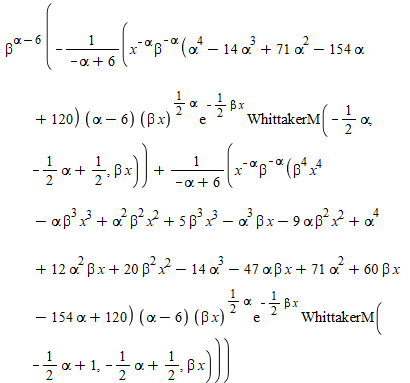Maple 2018

Good day,